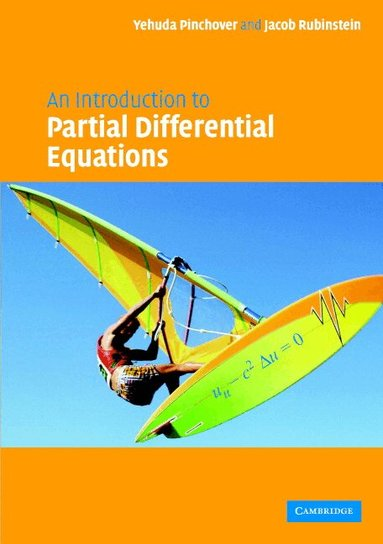Vetenskap & teknik

# An Introduction to Partial Differential Equations

## Jacob Rubinstein•Yehuda Pinchover

629:- (789:-)

Funktionen begränsas av dina webbläsarinställningar (t.ex. privat läge).

Hos dig inom 7-11 arbetsdagar

Fri frakt vid köp över 149:- för medlemmar.

• 381 sidor
• 2005
A complete introduction to partial differential equations, this textbook provides a rigorous yet accessible guide to students in mathematics, physics and engineering. The presentation is lively and up to date, paying particular emphasis to developing an appreciation of underlying mathematical theory. Beginning with basic definitions, properties and derivations of some basic equations of mathematical physics from basic principles, the book studies first order equations, classification of second order equations, and the one-dimensional wave equation. Two chapters are devoted to the separation of variables, whilst others concentrate on a wide range of topics including elliptic theory, Green's functions, variational and numerical methods. A rich collection of worked examples and exercises accompany the text, along with a large number of illustrations and graphs to provide insight into the numerical examples. Solutions to selected exercises are included for students and extended solution sets are available to lecturers from solutions@cambridge.org.
• Författare: Jacob Rubinstein, Yehuda Pinchover